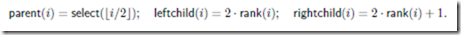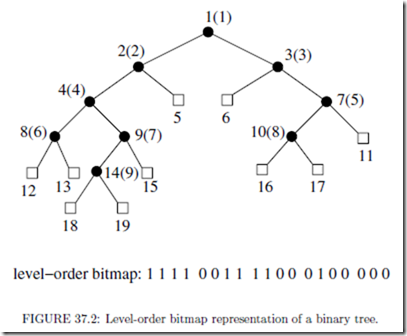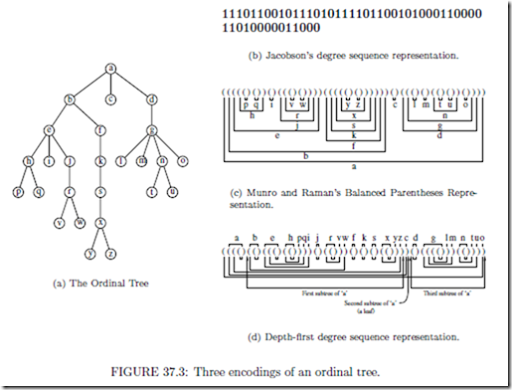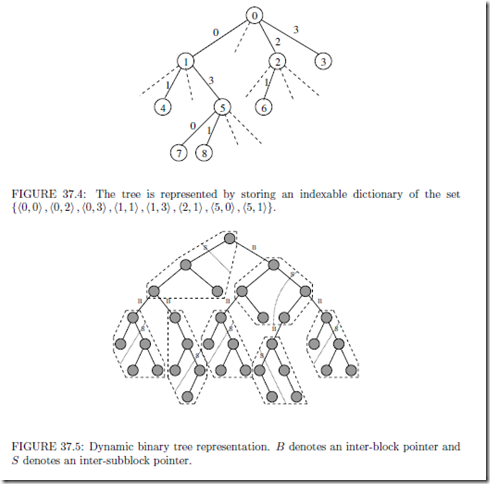# Succinct Representation of Data Structures:Tree Representations.

Tree Representations

Trees are one of the most fundamental objects in computer science. We consider the problem of representing large trees succinctly. Storing a tree with a pointer per child as well as other structural information can account for the dominant storage cost. For example, standard representations of a binary tree on n nodes, using pointers, take O(n lg n) bits of space.

Since there are only (2n+1//(2n + 1) diﬀerent binary trees on n nodes, less than 2n bits suﬃce to distinguish between them. We look at some binary tree representations that take 2n + o(n) bits and support the basic navigational operations in constant time.

Binary Trees

First, if the tree is a complete binary tree (i.e., a binary tree in which every level, except possibly the deepest, is completely ﬁlled, and the last level is ﬁlled from the left as far as required), then there is a unique tree of a given size and we require no additional space to store the tree structure. In fact, by numbering the nodes from 1 to n in the ‘heap order’  (left-to-right level-order traversal of the tree), one can support navigational operations on the tree by observing that the parent of a node numbered i is the node numbered li/2J, and the left and right children of node i are 2i and 2i + 1 respectively. But this property does not hold when the tree is not complete.

If the tree is not complete, one could extend it to a complete binary tree with the same height and store a bit vector indicating which nodes are present in the tree (in the heap order of the complete tree) to support the operations eﬃciently. But this takes space exponential in the number of nodes, in the worst case.

To save space, one can use the following compressed representation due to Jacobson :

First, mark all the nodes of the tree with 1 bits. Then add external nodes to the tree, and mark them with 0 bits. Construct a bitvector by reading oﬀ the bits that are marking the nodes in left-to-right level-order. (See Figure 37.2.) It is easy to see that the original tree can be reconstructed from this bitvector. For a binary tree with n nodes, this bitvector representation takes 2n + 1 bits. Moving between parent and child is just a slight twist on the method used in a heap. By storing the rank and select directories for this bitvector, one can support the navigational operations in constant time using the following equations:Ordinal Trees

Now, consider optimal representations of trees of higher degree, of which there are two diﬀerent notions.

An ordinal tree is a rooted tree of arbitrary degree in which the children of each node are ordered. Ordinal trees on n nodes are in one to one correspondence with binary trees on n nodes. Hence about 2n bits are necessary to represent an arbitrary ordinal tree on n nodes. A cardinal tree of degree k is a rooted tree in which each node has k positions for an edge to a child. Hence, a binary tree is a cardinal tree of degree 2. There are Ck (kn+1//(kn + 1) cardinal trees of degree k on n nodes . Hence we need roughly (lg(k 1) + k lg k )n k1 bits to represent an arbitrary such tree.

The basic operations we would like to support on tree representations are: given a node, ﬁnding its parent, i-th child, degree and the size of the subtree rooted at that node (subtree size). For the cardinal trees we also need to support the additional operation of ﬁnding a child with a given label.We outline three diﬀerent representations of an ordinal tree. All the three representations map the n nodes of the tree onto the integers 1,... , n, and hence all are appropriate for applications in which data is to associated with nodes or leaves.

Level-order unary degree sequence representation: A rooted ordered tree can be represented by storing its degree sequence in any of a number of standard orderings of the nodes. The ordinal tree encoding of Jacobson  represents a node of degree d as a string of d 1s followed by a 0. Thus the degree of a node is represented by a binary preﬁx code. These preﬁx codes are then written in a level-order traversal of the entire tree. Using auxiliary structures to support rank and select operations on this sequence, one can support ﬁnding the parent, the i-th child and the degree of any node in constant time. Thus, it gives a representation that takes 2n + o(n) bits of space and supports the above three operations in constant time, for an ordered tree on n nodes.

Balanced parenthesis representation: The tree encoding of Munro and Raman  uses a balanced sequence of parentheses to represent an ordinal tree. This balanced representation is derived from the depth-ﬁrst traversal of the tree, writing an open parenthesis on the way down and a close parenthesis on the way up. Thus, a tree on n nodes can be represented by a balanced parenthesis sequence of length 2n. Extending the ideas of Ja- cobson, they showed how to support the following operations in O(1) time, using negligible extra space (o(n) bits):

• ﬁndopen/ﬁndclose(i): ﬁnd the position of the open/close parenthesis matching the given close/open parenthesis in position i.

• excess(i): ﬁnd the diﬀerence between the number of open and closing parentheses

before the position i.

• enclose(i): given a parenthesis pair whose open parenthesis is in position i, ﬁnd

the open parenthesis corresponding to its closest enclosing matching parenthesis pair.The parent of a node can be found in constant time using the enclose operation. In the parenthesis representation, the nodes of a subtree are stored together, which enables us to support the operation of ﬁnding the size of the subtree rooted at a given node in constant time. The problem with this representation is that ﬁnding the i-th child takes Θ(i) time.

Depth-ﬁrst unary degree sequence representation: Jacobson’s representation al- lows access to the i-th child in constant time, whereas Munro and Raman’s representation supports subtree size operation in constant time. To combine the virtues of these two representations, Benoit et al.  used a representation that writes the unary degree sequence of each node in the depth-ﬁrst traversal order of the tree. The representation of each node contains essentially the same information as in Jacobson’s level-order degree sequence, but written in a diﬀerent order. Thus, it gives another 2n bit encoding of a tree on n nodes. Replacing the 0’s and 1’s by open and close parentheses respectively, and adding an extra open parenthesis at the beginning, creates a string of balanced parentheses. Using auxiliary structures to support rank and select operations on this bit string and also the operations on balanced parenthesis sequences deﬁned above, one can support ﬁnding the parent, i-th child, degree and subtree size of a given node in constant time.

Other operations: Sadakane  has shown that the parenthesis representation of an ordinal tree can be used to support least common ancestor queries in O(1) time using a o(n)-bit auxiliary structure. Munro and Rao  have shown that one can also support the level ancestor queries in O(1) time, using an additional o(n) bit auxiliary structure by storing the parenthesis representation. Geary et al.  obtained another structure that takes 2n + o(n) bits and supports level-ancestor queries, in addition to all the other navigational operations mentioned above in O(1) time.

Cardinal Trees

A simple cardinal tree encoding can be obtained as follows: Encode each node of a k-ary tree by k bits, where the ith bit speciﬁes whether child i is present. These can be written in any ﬁxed ordering of the tree nodes, such as level order or depth-ﬁrst order, to obtain the tree encoding. By storing the rank and select directories for this bitvector encoding, one can support parent, i-th child and degree queries in constant time. This encoding has the major disadvantage of taking kn bits, far from the lower bound of roughly (lg k + lg e)n bits, as there are Ck (kn+1//(kn + 1) k-ary cardinal trees on n nodes.

Using some probabilistic assumptions, Darragh et al.  have implemented a structure that takes lg k + O(1) bits per node, though the implementation treats lg lg n as ‘a constant’ (indeed 5). This structure supports the navigational operations in constant expected time and also supports updates ‘eﬃciently’ (compared with other linear space representations), and was also shown to perform well in practice.

To achieve a better space bound with good worst-case performance, one can use the ordinal tree encoding to store the underlying tree, and store some additional information about which children are present at each node. The ordinal information (using the depth- ﬁrst unary degree sequence representation) can be used to support the parent, i-th child, degree and subtree size queries in constant time.

Let Sx = {i1, i2,…, id} be the child labels of a node x with degree d in the cardinal tree.

To ﬁnd the child labeled j of node x, it suﬃces to ﬁnd i = rank(j) in the set Sx, if j Sx. If i = 1 (i.e., j /∈ Sx), then there is no child labeled j at node x, otherwise the i-th child of x is the child labeled j of node x. The i-th child can be found using the ordinal information.

Storing each of these sets Sx using the indexable dictionary representation of Section 37.3.1, which takes d lg k + o(d)+ O(lg lg k) bits for each Sx, requires n lg k + o(n)+ O(n lg lg k) bits in the worst case. Using a representation that stores a collection of indexable dictionaries eﬃciently , one can reduce the space consumption to n lg k + o(n) + O(lg lg k) bits.

Thus, this structure uses 2n + o(n) bits to represent the underlying ordinal tree, n lg k + o(n + lg k) bits to represent the labels of the children at each node, and supports all the navigational operations and the subtree size operation in O(1) time.

Using the succinct indexable dictionary structure mentioned in Section 37.3, Raman et al.  obtained an optimal space cardinal tree representation. The main idea is to store the set of all pairs, (i, j) such that the i-th node, in the level-order of the nodes, has a child labeled j, using an indexable dictionary representation.

(See Figure 37.4 for an example.)

Since

this set is of size n from the universe [nk], it requires lg (nk/ + o(n + lg k) = Ck + o(n + lg k) bits to store an indexable dictionary for this set. One can easily map the navigational operations on the tree to the operations on this set, to support them in constant time. But this structure does not support the subtree size operation eﬃciently.

Dynamic Binary Trees

All the tree representations mentioned so far are static. Even to make a minor modiﬁcation to the tree, such as adding a leaf, the entire structure has to be reconstructed (in the worst case). In this section we look at some representations that are more eﬃcient in supporting updates to the tree.

Munro et al.  gave a binary tree representation that takes 2n+ o(n) bits, and supports parent, left child, right child and subtree size operations in O(1) time. Updating the tree (adding a leaf or adding a node along an edge) requires O(lgc n) time, for some constantc 1 which depends on the size of the data associated with the nodes. Extending some of the ideas involved in this, Raman and Rao  improved the update time to O((lg lg n)E), for any ﬁxed E > 0, while maintaining the other time and space bounds.

We brieﬂy outline the key issues involved in the construction of these structures. First, we divide the tree into blocks of size Θ(lgc n), for some c 2, and each block in turn into sub-blocks of size E lg n, for some ﬁxed E < 1. The sub-blocks are stored using an implicit representation and are operated upon using precomputed tables. The block structure of the tree is stored using explicit pointers. Since there are only Θ(lgc1 n) sub-blocks in each block, we can store the sub-block structure within a block explicitly using Θ(lg lg n) sized pointers. Each block stores its parent block and the size, using a constant number of words. Thus, the overall block structure of the tree is easily handled by conventional means (storing explicit pointers) and only takes O(n/ lg n) bits. The blocks and sub-blocks tolerate some slack in their sizes and are moved to appropriate sized areas to avoid wasting space. Ultimately, the key issues boil down to the memory management.

To support subtree size, we maintain the the subtree sizes of the roots of all blocks and sub-blocks. Since each update changes the subtree sizes of several nodes, it is not possible to update all the eﬀected blocks and sub-blocks in constant time, in general. For this reason, we assume that the navigation through the tree begins at the root and may end at any point (or at the root, to achieve worst-case constant time for updates), and navigates the tree by moving from a node only to either its parent or one of its children. Hence, updates to a node higher in the tree regarding the insertions and deletions to descendants are made on return to that node.